# 全面支援主流模型稀疏化訓練及推理

## 覆蓋稀疏計算主流應用場景

### 3D點雲目標檢測

CenterPoint是一種物體檢測器，以點雲作為輸入，將三維物體在Bird-View下的中心點作為關鍵點，基於關鍵點檢測的方式迴歸物體的尺寸、方向和速度。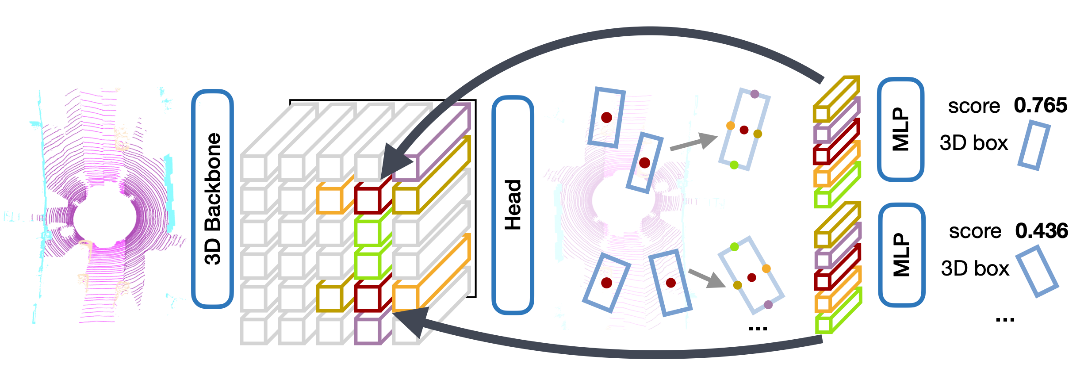• CenterPoint模型介紹

### Sparse Transformer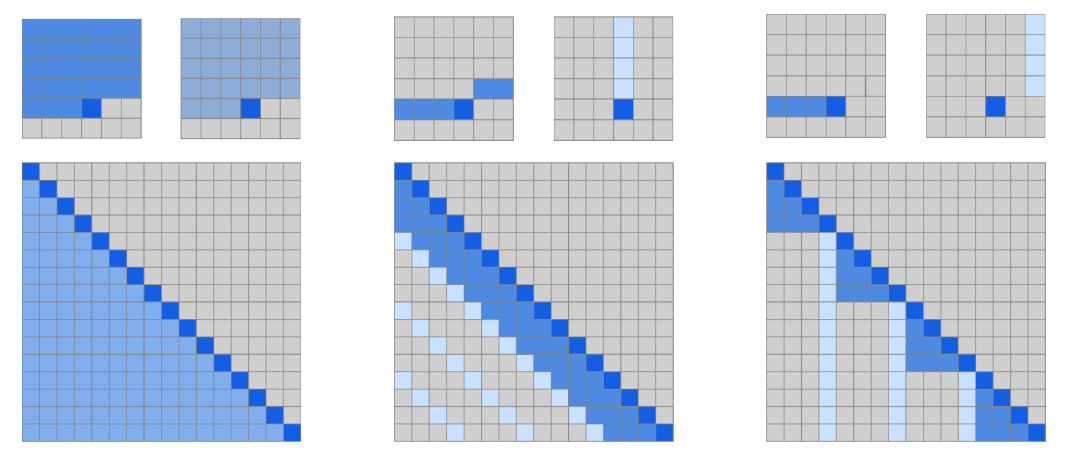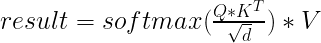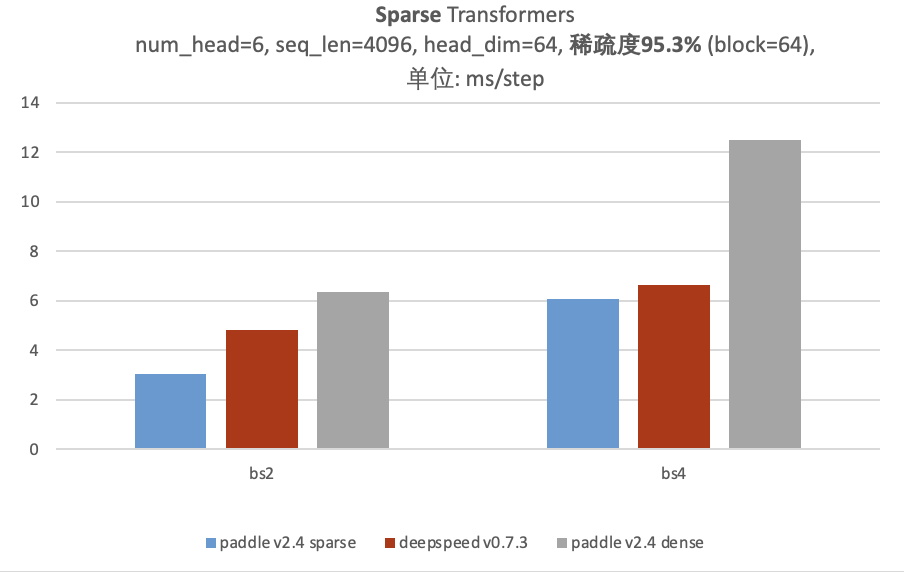## 支援多種稀疏Tensor格式及稀疏Tensor與稠密Tensor的混合計算

``````# 稀疏COO Tensor
indices = [[0, 1, 2],
[1, 2, 0]],
values  = [1., 2., 3.],
shape   = [3, 3])

# 稀疏CSR Tensor
crows  = [0, 1, 2, 3],
cols   = [1, 2, 0],
values = [1., 2., 3.],
shape  = [3, 3])
``````

``````# COO與Dense矩陣乘，返回稠密Tensor
indices = [[0, 1, 2],
[1, 2, 0]],
values  = [1., 2., 3.],
shape   = [3, 3])

# CSR與Dense矩陣乘，返回稠密Tensor
crows  = [0, 1, 2, 3],
cols   = [1, 2, 0],
values = [1., 2., 3.],
shape  = [3, 3])

# Dense與Dense矩陣乘，返回稀疏Tensor
crows = [0, 2, 3, 5],
cols  = [1, 3, 2, 0, 1],
values= [1., 2., 3., 4., 5.],
shape = [3, 4])
``````

## 名稱和使用方式與常規稠密Tensor的API保持一致，方便記憶且容易上手

``````import paddle

def __init__(
self,
in_channels,
out_channels,
stride=1,
downsample=None,
):
super(SparseBasicBlock, self).__init__()
self.conv1 = nn.SubmConv3D(
in_channels,
out_channels,
kernel_size=3,
stride=stride,
self.bn1 = nn.BatchNorm(out_channels, epsilon=1e-3, momentum=0.01)
self.relu = nn.ReLU()
self.conv2 = nn.SubmConv3D(
out_channels,
out_channels,
kernel_size=3,
stride=stride,
self.bn2 = nn.BatchNorm(out_channels, epsilon=1e-3, momentum=0.01)
self.downsample = downsample

def forward(self, x):
identity = x

out = self.conv1(x)
out = self.bn1(out)
out = self.relu(out)

out = self.conv2(out)
out = self.bn2(out)

if self.downsample is not None:
identity = self.downsample(x)

out = self.relu(out)

return out
``````

# 新增圖學習類API，支援高效圖學習計算

## 高效圖訊息傳遞

``````import paddle

x = paddle.to_tensor([[0, 2, 3], [1, 4, 5], [2, 6, 7]], dtype="float32")
y = paddle.to_tensor([1, 1, 1, 1], dtype="float32")
indexes = paddle.to_tensor([[0, 1], [1, 2], [2, 1], [0, 0]], dtype="int32")
src_index, dst_index = indexes[:, 0], indexes[:, 1]
``````

## 高效能圖取樣

``````import paddle

row = np.array([3, 7, 0, 9, 1, 4, 2, 9, 3, 9, 1, 9, 7])
colptr = np.array([0, 2, 4, 5, 6, 7, 9, 11, 11, 13, 13])
row = core.eager.to_uva_tensor(row)
colptr = core.eager.to_uva_tensor(colptr)
nodes = paddle.to_tensor([0, 8, 1, 2])
sample_size = 2
# 鄰居取樣API的輸入要求圖結構資訊為CSC格式
neighbors, neighbor_count = paddle.geometric.sample_neighbors(row, colptr, nodes, sample_size=sample_size)
# 生成重編號後的邊
reindex_src, reindex_dst, out_nodes = paddle.geometric.reindex_graph(nodes, neighbors, neighbor_count)
``````

# 新增語音領域類API

## 高效的特徵提取模組

``````import paddle

#設定音訊相關引數
sample_rate = 16000
wav_duration = 0.5
num_channels = 1
num_frames = sample_rate * wav_duration
wav_data = paddle.linspace(-1.0, 1.0, num_frames) * 0.1
waveform = wav_data.tile([num_channels, 1])

#設定特徵提起器相關引數
feature_extractor = LogMelSpectrogram(sr=sample_rate, n_fft=512, window = 'hann', power = 1.0)
feats = feature_extractor(waveform)
``````

## 音訊處理基礎模組

``````import paddle

#cosine窗函式示例
n_fft = 512

#高斯窗函式
std = 7

#離散餘弦變換示例
n_mfcc = 23
n_mels = 257
``````

## 語音IO模組

``````import os

#設定相關引數，生成示例音訊
sample_rate = 16000
wav_duration = 0.5
num_channels = 1
num_frames = sample_rate * wav_duration
wav_data = paddle.linspace(-1.0, 1.0, num_frames) * 0.1
waveform = wav_data.tile([num_channels, 1])
base_dir = os.getcwd()
filepath = os.path.join(base_dir, "test.wav")

#儲存和提取音訊資訊
#wav_info中會有sample_rate, num_frames, num_channels等資訊
``````

## 語音分類資料集

``````import paddle

mode = 'dev'
feat_type='raw')
for idx in range(5):
audio, label = esc50_dataset[idx]
# do something with audio, label
print(audio.shape, label)
# [audio_data_length] , label_id

feat_type='mfcc',
n_mfcc=40)
for idx in range(5):
audio, label = esc50_dataset[idx]
# do something with mfcc feature, label
print(audio.shape, label)
# [feature_dim, length] , label_id
``````

# 其它新增的API

## 分散式API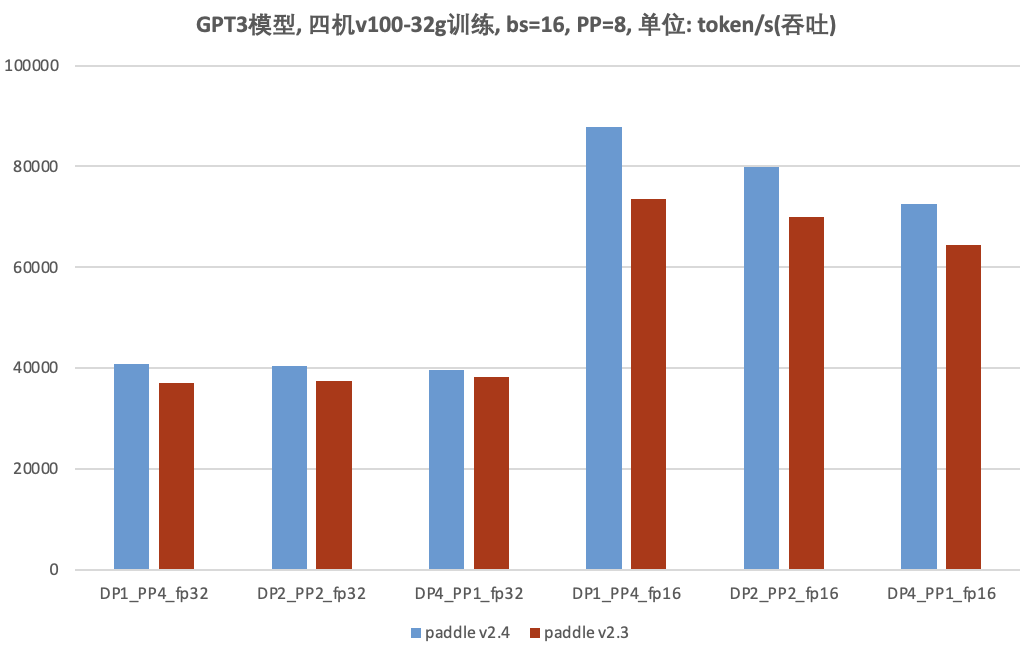## 視覺變換API

• Release Note地址

# 結語

• 簡化API開發步驟飛槳框架v2.4完成了基礎框架運算元體系重構，構造高可複用的PHI運算元庫(Paddle HIgh reusability operator library)，支援基於已有的運算元核心以及Kernel Primitives API組合實現新的運算元，支援外掛式接入新硬體或者新加速庫。PHI運算元庫的成熟，提升了飛槳API的開發效率，並形成了通用的API開發流程，使得開發者可以更加簡潔流暢地參與飛槳API的開發與貢獻。

• 釋出標準API貢獻指南飛槳框架v2.4形成了標準的API貢獻指南，包括貢獻流程與操作指南、API設計文件模板、API程式碼模板、API文件寫作規範，為飛槳社群開發者提供清晰的文件指引與輔助，使得開發者可以快速上手。

• 提供標準開發環境飛槳AIStudio平臺推出標準開發環境，為開發者提供飛槳映象環境、線上IDE與專屬GPU算力，登入即可開發除錯，免去環境配置與算力限制，隨時隨地參與飛槳框架的開發與貢獻。